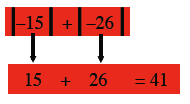### Home > ACC6 > Chapter 4 Unit 4 > Lesson CC1: 4.1.3 > Problem4-37

4-37.

Simplify each of the following absolute value expressions. Homework Help ✎

Absolute value is the distance a number is from zero on the number line in either direction.
We use the special symbol shown below to indicate the absolute value of any number x.

The absolute value of any number is non-negative.

$|-3|=3\text{ and }|3|=3$

1. $| - 15 | + | - 26 |$1. $- | - 40 |$

1. $| 0.5 | + | - 1 \frac { 1 } { 2 } |$